# MEDIAN Function

Calculates the middle value of a given set of numbers

## What is the MEDIAN Function?

The MEDIAN Function is categorized under Excel Statistical functions. The function will calculate the middle value of a given set of numbers. Median can be defined as the middle number of a group of numbers. That is, half the numbers return values that are greater than the median, and half the numbers return values that are less than the median. For example, the median of 2, 3, 3, 5, 7, and 10 is 4.

In financial analysis, the function can be useful in calculating the median of certain numbers, e.g., median sales or median expenses.

### Formula

=MEDIAN(number1, [number2], …)

The function uses the following arguments:

1. Number1 (required argument) – The number arguments are a set of one or more numeric values (or arrays of numeric values), for which we wish to calculate the median.
2. Number2 (optional argument)

For the MEDIAN function, remember that:

1. If there is an even number of values in the dataset, the average of the two middle values is returned.
2. If a supplied array contains empty cells, text, or logical values, these values are ignored by the function when calculating the median.
3. In current versions of Excel (Excel 2007 and later), you can provide up to 255 number arguments to the MEDIAN function. In Excel 2003, the function can only accept up to 30 number arguments. However, each of the number arguments can be an array of many values.

### How to use the MEDIAN Function in Excel?

As a worksheet function, MEDIAN can be entered as part of a formula in a cell of a worksheet. To understand the uses of the function, let us consider a few examples:

#### Example 1

Let’s understand how this function would calculate the median using the set of values below: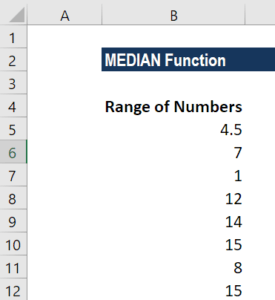Here we provided an odd set of values. The formula used was: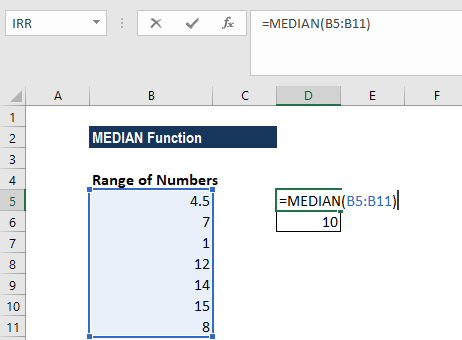We get the results below: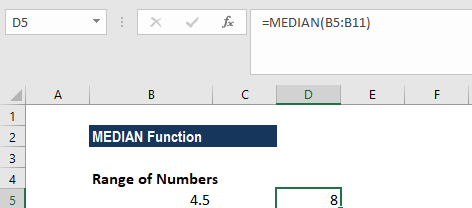Suppose we now provide an even set of numbers and use the formula below: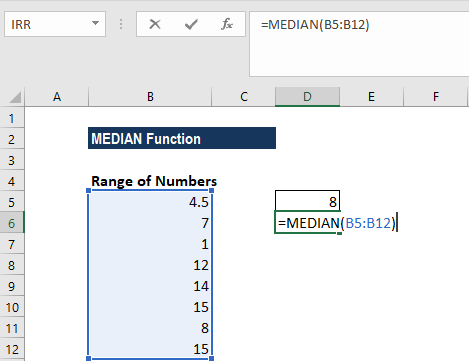As it is an even set of numbers, the average of the two middle values is returned.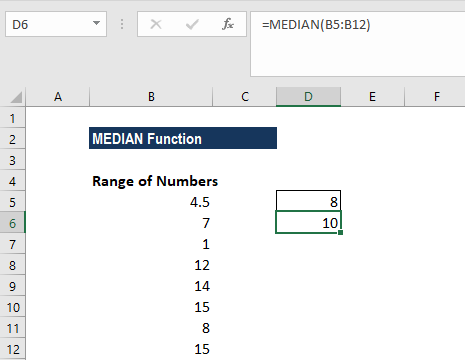#### Example 2

For the data below: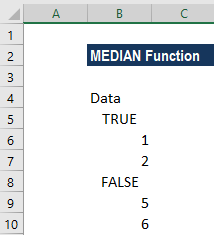We used the following formula: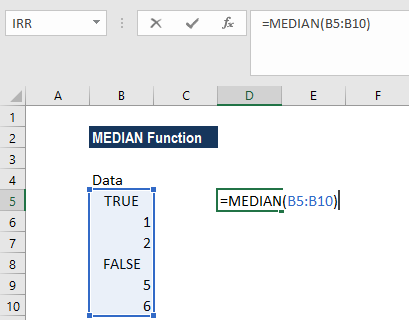While calculating the median for the above range, the function ignored the logical values and gave the result below: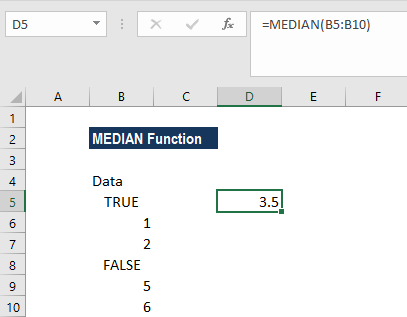Thanks for reading CFI’s guide to important Excel functions! CFI is the official provider of the Financial Modeling & Valuation Analyst certification.

By taking the time to learn and master these functions, you’ll significantly speed up your financial analysis. To learn more, check out these additional CFI resources:

• Excel Functions for Finance